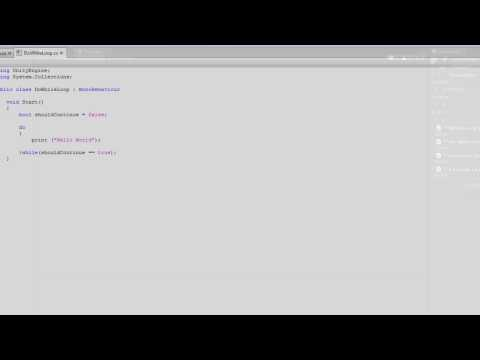# Loops

#### 난이도: 초급

How to use the For, While, Do-While, and For Each Loops to repeat actions in code.## Loops

초급 Scripting

### Code snippet

``````using UnityEngine;
using System.Collections;

public class ForLoop : MonoBehaviour
{
int numEnemies = 3;

void Start ()
{
for(int i = 0; i < numEnemies; i++)
{
Debug.Log("Creating enemy number: " + i);
}
}
}``````
``````#pragma strict

var numEnemies : int = 3;

function Start ()
{
for(var i : int = 0; i < numEnemies; i++)
{
Debug.Log("Creating enemy number: " + i);
}
}``````
``````import UnityEngine
import System.Collections

public class ForLoop(MonoBehaviour):

private numEnemies = 3

private def Start():
for i in range(0, numEnemies):
Debug.Log(('Creating enemy number: ' + i))``````

### Code snippet

``````using UnityEngine;
using System.Collections;

public class WhileLoop : MonoBehaviour
{
int cupsInTheSink = 4;

void Start ()
{
while(cupsInTheSink > 0)
{
Debug.Log ("I've washed a cup!");
cupsInTheSink--;
}
}
}``````
``````#pragma strict

var cupsInTheSink : int = 4;

function Start ()
{
while(cupsInTheSink > 0)
{
Debug.Log ("I've washed a cup!");
cupsInTheSink--;
}
}``````
``````import UnityEngine
import System.Collections

public class WhileLoop(MonoBehaviour):

private cupsInTheSink = 4

private def Start():
while cupsInTheSink > 0:
Debug.Log('I\'ve washed a cup!')
cupsInTheSink -= 1``````

### Code snippet

``````using UnityEngine;
using System.Collections;

public class DoWhileLoop : MonoBehaviour
{
void Start()
{
bool shouldContinue = false;

do
{
print ("Hello World");

}while(shouldContinue == true);
}
}``````
``````#pragma strict

function Start()
{
var shouldContinue : Boolean = false;

do
{
print ("Hello World");

}while(shouldContinue == true);
}``````
``````import UnityEngine
import System.Collections

public class DoWhileLoop(MonoBehaviour):

private def Start():
shouldContinue = false

while true:
print('Hello World')
break  unless (shouldContinue == true)``````

### Code snippet

``````using UnityEngine;
using System.Collections;

public class ForeachLoop : MonoBehaviour
{
void Start ()
{
string[] strings = new string;

strings = "First string";
strings = "Second string";
strings = "Third string";

foreach(string item in strings)
{
print (item);
}
}
}``````
``````#pragma strict

function Start ()
{
var strings = ["First string", "Second string", "Third string"];

for(var item : String in strings)
{
print (item);
}
}``````
``````import UnityEngine
import System.Collections

public class ForeachLoop(MonoBehaviour):

private def Start():
strings as (string) = array(string, 3)

strings = 'First string'
strings = 'Second string'
strings = 'Third string'

for item as string in strings:
print(item)``````

#### 관련 자습서